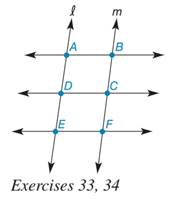Chapter 5.2, Problem 34EElementary Geometry For College St...

7th Edition
Alexander + 2 others
ISBN: 9781337614085

Solutions

Chapter
SectionElementary Geometry For College St...

7th Edition
Alexander + 2 others
ISBN: 9781337614085
Textbook Problem

In the drawing, A B ↔   | |     D C ↔     | |       E F ↔ . Suppose that transversals l and m are also parallel. D and C are the midpoints of A E ¯ and B F ¯ , respectively. If parallelogram ABCD similar to parallelogram DCFE?To determine

To check:

Whether the parallelogram ABCD is similar to DCFE or not

Explanation

Given:

In the figure,

AB||DC||EF with transversals l and m. If D and C are the midpoints of AE¯ and BF¯.

Approach:

Two trapezoids are similar if and only if two conditions are satisfied:

1. Three or more parallel lines intercept congruent line segments on one transversal.

2. The intercept congruent line segments on any transversal.

Proof:

Let us consider the parallelograms, ABCD and DCFE.

Since AB||DC||EF, all pairs are parallel lines, intercept congruent line segments on one transversal.

That is, if two or more parallel lines intersect two transversals, l and m, then the parallel lines cut off the transversals proportionally

Still sussing out bartleby?

Check out a sample textbook solution.

See a sample solution

The Solution to Your Study Problems

Bartleby provides explanations to thousands of textbook problems written by our experts, many with advanced degrees!

Get Started

Fill in each blank: 9000lb=tons

Elementary Technical Mathematics

In Exercises 13-20, sketch a set of coordinate axes and plot each point. 14. (1, 3)

Applied Calculus for the Managerial, Life, and Social Sciences: A Brief Approach

Find the limit. limx3x+6xx33x2

Single Variable Calculus: Early Transcendentals, Volume I

For y = 3x, y = a) 3x log3 e b) 3x ln 3 c) 3xlog3e d) 3xln3

Study Guide for Stewart's Single Variable Calculus: Early Transcendentals, 8th

It does not exist.

Study Guide for Stewart's Multivariable Calculus, 8th